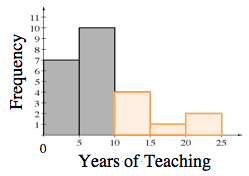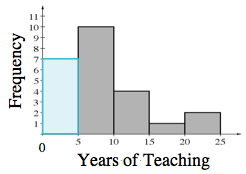### Home > MC2 > Chapter 10 > Lesson 10.2.1 > Problem10-89

10-89.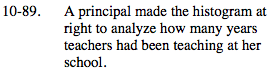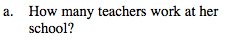Add up the frequencies represented by the bars. How many total teachers are there?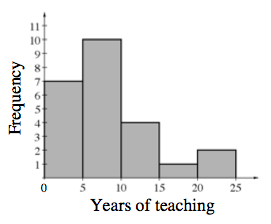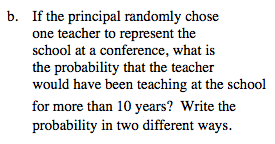Based on the histogram, how many teachers have been teaching for more than 10 years?

How can this probability be represented as a fraction and a percent?

$\frac{7}{24}\text{ or}\approx29 \%$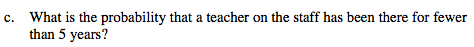Based on the histogram, how many teachers have been teaching for less than 5 years?